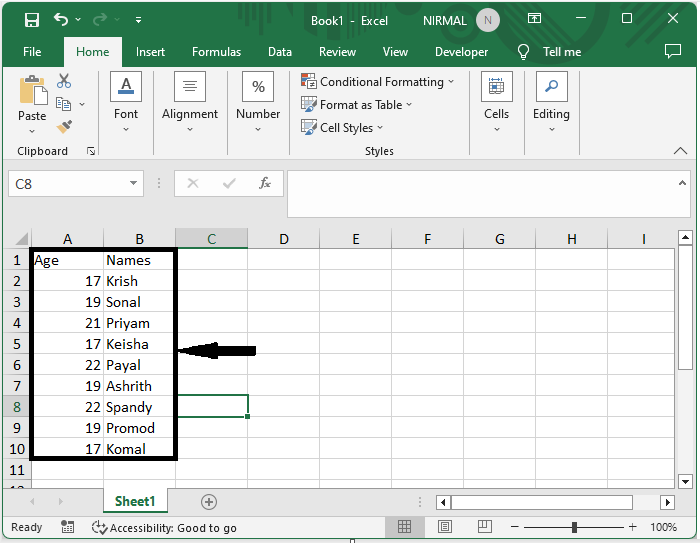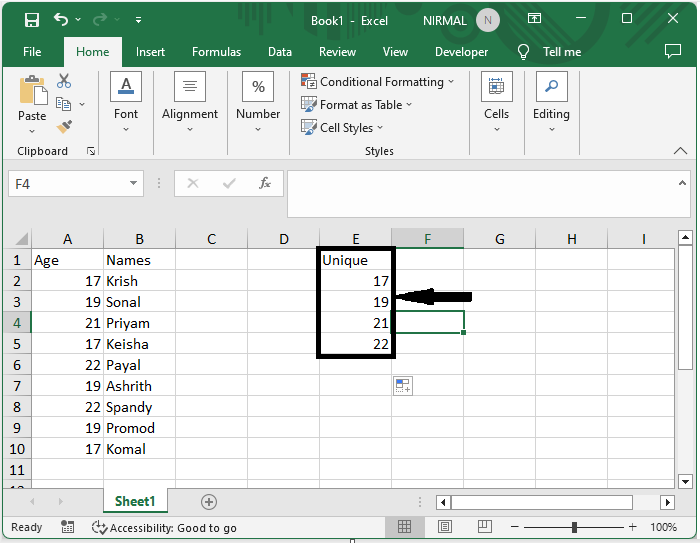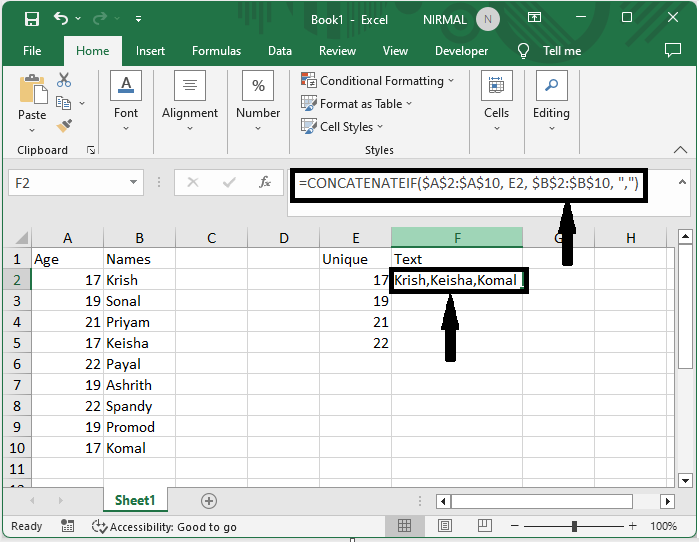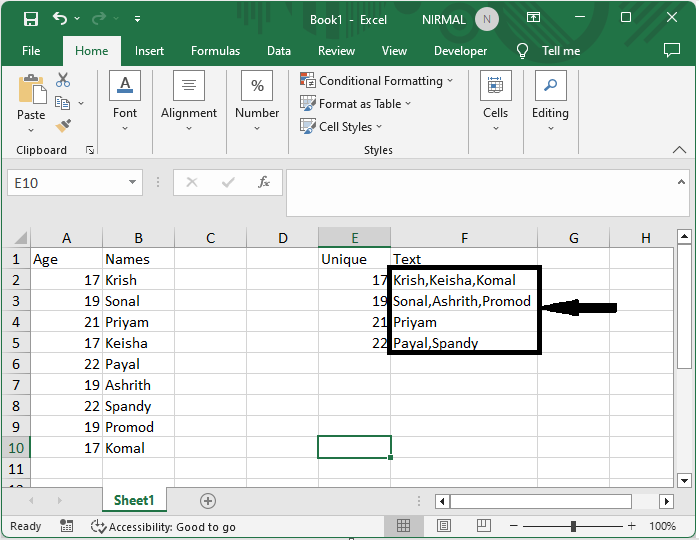# How To Easily Concatenate Text Based On Criteria In Excel?

When you wish to merge different pieces of information into a single cell or column, concatenating text in Excel is a regular activity. However, there are instances when you need to concatenate text based on specific criteria or conditions, and this tutorial can help. By the end of this article, you will have learned essential tips and tactics for streamlining your text concatenation chores and improving the efficiency of your Excel experience. So, if you're ready to advance your Excel skills and master the art of concatenating text depending on criteria, let's get started!

## Easily Concatenate Text Based On Criteria

Here we will first list the unique values, then create a user−defined formula using VBA and use the formula to complete the task. So let us see a simple process to know how you can easily concatenate text based on criteria in Excel.

### Step 1

Consider an Excel sheet where the data in the sheet is similar to the below image.First to list the unique values, click on an empty cell and enter the formula as =IFERROR(INDEX($A$2:$A$15, MATCH(0,COUNTIF($D$1:D1, $A$2:$A$15), 0)), and click Ctrl + Shift + Enter. Then drag down using the autofill handle.

Empty cell > Formula > Ctrl + Shift + Enter.### Step 2

Now to create a user−defined formula, right−click on the sheet name and select view code to open a VBA application, then click on insert and select module.

Right click > View code > Insert > Module.

### Step 3

Then copy the below code into the text box.

### Code

Function ConcatenateIf(CriteriaRange As Range, Condition As Variant, ConcatenateRange As Range, Optional Separator As String = ",") As Variant
Dim xResult As String
On Error Resume Next
If CriteriaRange.Count <> ConcatenateRange.Count Then
ConcatenateIf = CVErr(xlErrRef)
Exit Function
End If
For i = 1 To CriteriaRange.Count
If CriteriaRange.Cells(i).Value = Condition Then
xResult = xResult & Separator & ConcatenateRange.Cells(i).Value
End If
Next i
If xResult <> "" Then
xResult = VBA.Mid(xResult, VBA.Len(Separator) + 1)
End If
ConcatenateIf = xResult
Exit Function
End Function


### Step 4

Then use Alt+Q to close the VBA. Then click on an empty cell, in this case cell E2, and enter the formula as =CONCATENATEIF($A$2:$A$15, D2, $B$2:$B$15, ",").

Alt + Q > Empty cell > Formula > Enter.### Step 5

Then drag down using the autofill handle to complete the task.## Conclusion

In this tutorial, we have used a simple example to demonstrate how you can easily concatenate text based on criteria in Excel to highlight a particular set of data.

Updated on: 13-Jul-2023

56 Views